Dr. P.V. Viswanath
 Home Bio Courses Research Economics/Finance on the Web Student InterestCourses/Classnotes# Options ©P.V. Viswanath, 1997, 2007

### The Binomial Model

Consider the following security: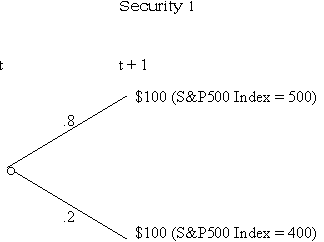Note that this security has no risk. Assuming an equilibrium rate of return of 8% on other assets with zero risk, the appropriate discount rate in this case should yield a price/present value of 100/1.08 = 92.59.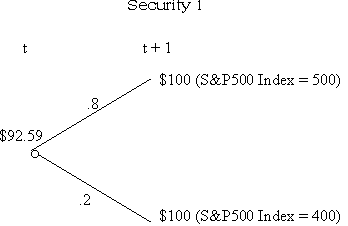Now, let's look at another case.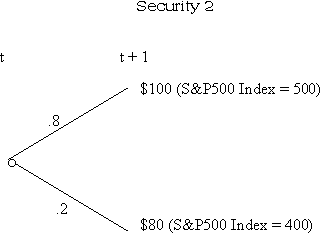What is the present value in this case? Let us assume that the S&P 500 Index represents the market portfolio. Then, since the asset covaries perfectly with the market index, the discount rate is simply the equilibrium rate of return on the market portfolio. Let's assume that this is 12%. In this case, we take the expected value of the payoff on the security, and discount it back to period t at 12%, which in this case, works out to \$85.71.

Now what about the following case:The expected cash flow in period t+1 is \$98. If we discount this at 8%, the present value is \$90.74; if we discount it at 12%, the answer is \$87.50. But which is the correct answer?

### Using the Arbitrage Principle for valuation

Security 3's cashflows are a simple average of the cash flows of securities 1 and 2 in the 2 scenarios (states of the world). Hence, the value of security 3 is also a simple average of the values of securities 1 and 2. The present value of security 3 is 0.5(92.59) + 0.5(85.71) = 89.15. If we work out the expected rate of return that is consistent with this price, we see that it is [0.8(100)+0.2(90)]/89.15 - 1 or 9.9271%. But where does this strange discount rate come from? We'll see a little bit later. But first, some important information about using the arbitrage principle for valuation.

Note that we did not need to know the probabilities of the two states, once we had the prices of the riskfree asset and the S&P portfolio. This is because we are using the principle of no riskless arbitrage. For the application of this principle, we do not need to know probabilities. This principle states:

If the cashflows on an asset can be expressed as a weighted average of the cashflows on a given set of traded assets, the market price of the first asset must be equal to the same weighted average of the market prices of the given set of traded assets. If this is not so, then there will be opportunities for riskless arbitrage profits.

But once we have this idea of a security being reckoned in terms of an average of other securities, we can take this further. Take security 4.Can security 4 be considered a weighted average of securities 1 and 2? Let's conjecture that this is indeed possible. Then, let the weights be l and s. In this case, it must be true that

l(Cashflow in state 1 for sec. 1) + s(Cashflow in state 1 for sec. 2) = 10;

l(Cashflow in state 2 for sec. 1) + s(Cashflow in state 2 for sec. 2) = 0, which gives us the two simultaneous equations:

100l + 100s = 10

100l + 80s = 0

Solving these equations simultaneously, we get s = 0.5, and l = -0.4. Reconstituting the cashflows, we see that 100 (-0.4) + 100 (0.5) = 10 in state 1, and 100 (-0.4) + 80 (0.5) = 0 in state 2, as required. But this implies that the value of security 4 is simply (-0.4) (92.59) + (0.5) (85.71) = \$5.82.

Let's go back to Security 3, now. The corresponding equations in this case are:

100l + 100s = 100

100l + 80s = 90

Solving these equations simultaneously, we get s = 0.5, and l = 0.2. Reconstituting the cashflows, we see that 100 (0.5) + 100 (0.5) = 100 in state 1, and 100 (0.5) + 80 (0.5) = 90 in state 2, as required. But this implies that the value of security 4 is simply (0.5) (92.59) + (0.5) (85.71) = \$89.15, which we have already seen.

The interesting thing that we have derived in addition to what we had before is that security 4 is equivalent to a portfolio consisting of a 50% investment in the market portfolio and a 50% investment in the risk-free asset. Hence its beta is 0.5(1)+0.5(0) = 0.5. According to the CAPM, then it's expected rate of return is 8 + 0.5(12-8) = 10%, which is pretty much the expected rate of return of 9.9271% that we got through the use of the arbitrage principle. (If you're wondering why there's a discrepancy between the 9.9271% and the 10%, it's because the CAPM really holds in continuous time. Hence exp[ln((1.12)+ln(1.08))/2] = 1.1, which is the expected return that the CAPM gave us.)

### Introduction

An American option is a contract that gives its owner the right to sell (or buy) an underlying asset at a pre-specified price (exercise or strike price) within a specified period of time (option duration). An option to buy is called a call option and an option to sell is called a put option. A European option does not allow its owner to buy/sell the underlying asset until the specified period of time (expiration or maturity date) ends.

The hypothetical value of an option if it were assumed to be expiring immediately is called its intrinsic value. This is the difference between the current market value of the underlying asset and the exercise price. The difference between the actual value of an option and its intrinsic value is called its time value.

An option whose exercise price is greater than the current market value of the underlying asset is said to be out-of-the-money, while it is said to be in-the-money if the underlying asset is trading higher than the exercise price. If the two are the same, the option is at-the-money.

An option that is traded on an exchange is an exchange-traded option, while those traded, but not on an exchange are called over-the-counter option.

An option is an example of a larger class of securities whose values depend on the value of another underlying security. Such securities are called contingent claims or derivatives.

### Valuing Call Options

Suppose we have a call option on the portfolio of S&P 500 index stocks with an exercise price of \$490. If the index (portfolio) value at time t+1 is \$500, then the value of the call option is exactly \$10, since we can buy the index portfolio for \$490 and sell it for \$500. If the index value at time t+1 is \$400, then the call option is clearly worthless. We can graph this relation, using a payoff diagram (which shows the payoff to holding a certain asset or following a certain strategy as a function of another underlying asset's value):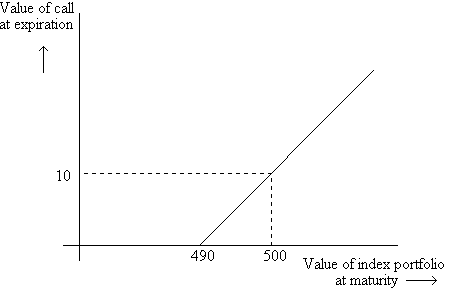Assume that only the values \$400 and \$500 are possible for the index portfolio at time t+1. Then, if we draw the binomial tree for this call option, we see that the cashflows are indeed the same as for security 4, and hence its value is the same.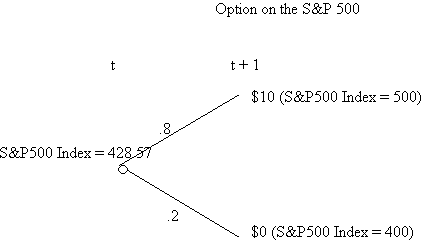The replicating portfolio of the index and the riskfree asset can be computed by solving the equations:

100l + 500s = 10

100l + 400s = 0

The solution is l = -0.4, and s = 0.1. The option value, as we remarked above is \$5.82.

The replicating portfolio approach has an alternative interpretation. Note that the value of the option is l[PV(100)] + s(the current price of the underlying asset). In other words, the price of the option is equal to the current market value of a portfolio consisting of s units of the underlying asset and l units of a bond paying \$100 at time t+1. We see, in this case, that the option is equivalent to a portfolio long 0.1 units of the underlying asset and short 0.4 units of the riskfree asset. Keeping in mind that being short the riskfree asset is equivalent to borrowing, we note that a call option can be replicated by simultaneously going long the underlying asset and borrowing.

Options that are written on an underlying index, such as the option that we just valued, are called index options.

### Determinants of Option Prices

The value of an option depends on several parameters and we need to know how changing these parameters, one at a time, affects the option value:

• the current asset price
• the exercise price
• the asset return volatility
• the rate of interest
• the time to maturity

We will use the previous example as our base example: a call option on the portfolio of S&P 500 index stocks with an exercise price of \$490.

#### Effect of changes in the asset return volatility:

The volatility in the return on an asset is usually measured by the standard deviation of the return on the asset. Let us increase the volatility of the return on the S&P 500 Index, keeping the mean return constant. For example, let us assume that the values of the index portfolio at time t+1 can be either 360 or 510. (Note that the expected value of the S&P 500 Index at time t+1 stays equal to 480.)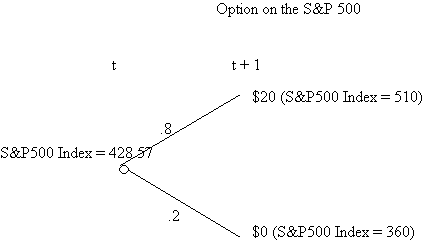The value of the option will go up because the value of the option in state 1 is now \$20, where it was formerly \$10. The value of the option in state 2 continues to be zero. The replicating portfolio will, of course, be different, but the net effect will still be to increase the value of the call option.

To confirm, we can compute the replicating portfolio. We must solve the equations:

100l + 510s = 20

100l + 360s = 0

Solving, we find l = -12/25, and s = 2/15. This gives us an option value of 12.70, which is greater than 5.82, as conjectured.

#### Effect of changes in the risk-free rate:

We have assumed a riskfree rate of 8%. If we increase the riskfree rate to 10%, the option (being equivalent to a portfolio long the stock and short the riskless asset) will increase in value, since the present value of the amount required at t+1 has dropped. The equations to be solved are the same as before, and hence the replicating portfolio stays the same.

100l + 500s = 10
100l + 400s = 0

However, the value of the option, now, is -0.4 (100/1.1) + 0.1 (428.57) = 6.49 > 5.82.

#### Effect of changes in the current asset price:

Let us now change the current price of the asset. Since we need to keep the return volatitliy constant, this can be achieved by changing the discount rate for the asset. Suppose the discount rate is 14% (instead of 12%). Then the stock price will drop, and the value of the option, which consists of a portfolio long in stocks will drop as well. The equations to be solved are the same as before, and hence the replicating portfolio stays the same.

100l + 500s = 10
100l + 400s = 0

However, the value of the option, now, is -0.4 (100/1.08) + 0.1 (480/1.14) = 5.07 < 5.82.

#### Effect of changes in the exercise price:

If we change the exercise price to 480 instead of 490, the equations to be solved change to:

100l + 500s = 20
100l + 400s = 0

The solution to these equations is: = -0.8; = 0.2; hence the option value is -0.8 (100/1.08) + 0.2 (480/1.12) = 11.64 > 5.82.

Intuitively, this is clear, because the higher the exercise price, the lower the upside potential of the option.

### Multi-period extensions of the binomial model

Let us assume that there is yet another period before the option matures. Now, from date t to t+1, the stock price has been assumed to either increase by 16.67% (from 428.57 to 500) or decrease by 6.67% (from 428.57 to 400). Let us assume that during the next period too, only the same kind of increase or decrease is possible. Hence the scenario can be shown as follows:In this instance, we need to compute the value of the call option at time t in two stages. We first compute the value of the option at time t+1 conditional on the index value. In other words, we compute what the call option value would be at t+1 if the index value were 500 or 400. Then we compute the option value at t, given the option values at t+1. This can be done in exactly the same way as we did it before.

Using this technique, we solve the equations:

100l + 583.33s = 93.33 and 100l + 466.66s = 0

to obtain the value of the call option at t+1 if the index value is 500.

Ans: l = -3.73; s = 0.8; call value = -3.73(100/1.08) + (0.8)(500) = 54.63.

If the value of the index value at t+1 is 400, the equations to solve are:

100l + 466.66s = 0 and 100l + 373.33s = 0

This gives us l = 0 and s = 0, or a call option value of 0.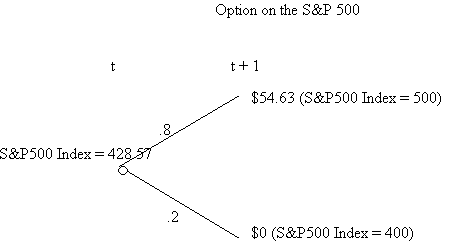The solutions to solve, in this case, are:

100l + 500s = 54.63 and 100l + 400s = 0

Solving, we get l = -2.185; s = 0.5463, for an option value of 31.81 > 5.82.

The reason that a greater time to maturity increases the value of the option is simply that the upside potential is greater, while the downside potential is always fixed.

 Parameter Effect the current asset price positive the exercise price negative the asset return volatility positive the rate of interest positive the time to maturity positive

### Pricing Options using the Black-Scholes method

The Black-Scholes formula gives the value, C, of a call option with exercise price E and expiring in t years, written on an asset with variance of the continuous return equal to s2 per year and currently selling at S. (This formula assumes that there is continuous trading, and that the option can only be exercised at maturity):

C = S N(d1) - E e-rt N(d2);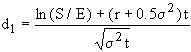and d2 = d1 -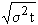N(.) is the area under the standardized normal curve (i.e. the plot of probability against value for a normally distributed variable with mean zero and s.d. = 1), and can be obtained by consulting normal probability tables.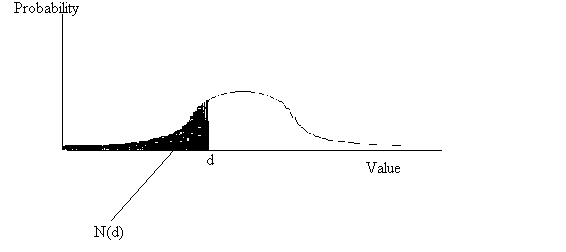Example:

Consider a call option with exercise price 490 and time to maturity equal to 1 year, on a stock selling at \$428.57, with a variance of 0.01245 per year. The riskless rate of return is 8% per year. What is the value of the option?

d1 = -0.4277; d2 = -0.5393; N(d1) = 0.3345 and N(d2) = 0.2946.

C = 428.57 (0.3345) - 490 (.9231) (.2946) = 10.10

### Put Options and Put-Call Parity

A put option on an asset is the right (but not the obligation) to sell a given quantity of that asset at a fixed (exercise) price within a fixed time period.

Example: Suppose we are considering a put option on the portfolio of S&P 500 index stocks with an exercise price of \$490. If the index (portfolio) value at time t+1 is \$500, then the value of the put option is worthless. If the index value at time t+1 is \$400, then the put option is worth exactly \$90, since we can buy the index portfolio for \$400 and sell it for \$490. We can graph this relation:A person who sells a call option (with an exercise price of \$490) and a person who sells a put option with an exercise price of \$490 will, however, have the following payoffs: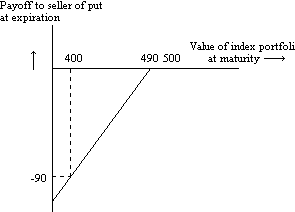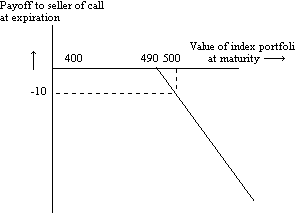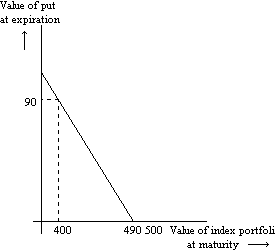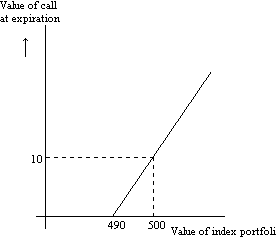We can also graph the payoff to the holder of one unit of an asset as a function of the asset price at some time in the future.Now consider combining a long position in the index portfolio along with a put on the index portfolio.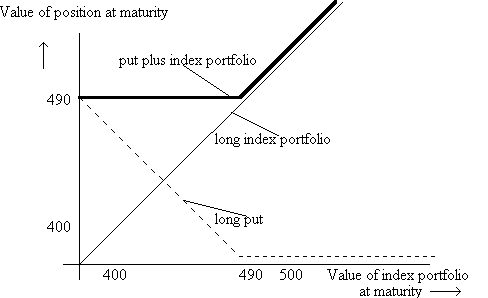Finally, let us combine a call with an investment in riskless bonds maturing at the same time as the call.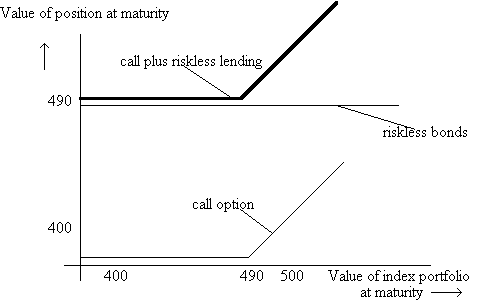The payoff at expiration of the two combinations is the same. Hence, the present value of the two combinations must also be the same. In general:

C + E e-rt = P + S, where P is the price of the put.

Example: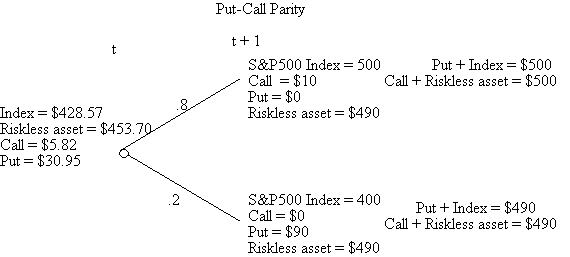We can graph the value of the call option before maturity as a function of the underlying asset price. In this case, the graph line will no longer be linear.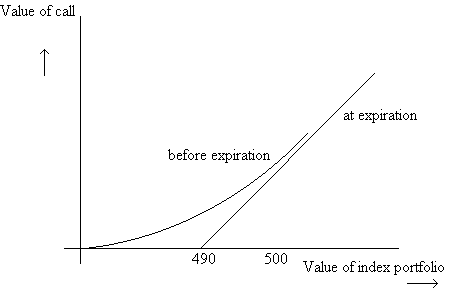### Stocks and Bonds as Options

We can explain some of the agency problems of debt by looking at the equity in the firm as a call option on the assets of the firm. Consider what happens to the equityholders of a firm. If the firm does well, they pay off the bondholders and they get the entire upside. If the firm is not doing well, they don't have to payoff the bondholders in full; they can just walk away from the firm. Hence, what they have is similar to an option.

We know that the value of an option is increasing in the volatility of the return on the underlying asset. Similarly, the management of a firm can increase the value of equity by increasing the riskiness (volatility) of returns on the firm's projects.

Example: Consider a firm with a project currently worth \$100. Next period, if the project succeeds, the firm will be worth \$200; else the value of the firm will drop to \$40. Suppose the firm currently has debt with a promised payment of \$60 next period.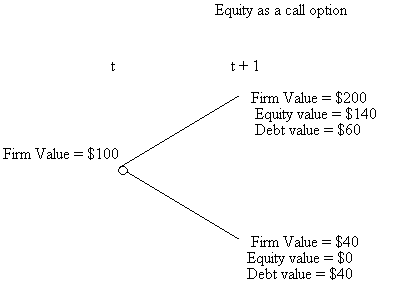Assuming an interest rate of 5%, the equations to be solved are:

200s + 1.05 L = 140 and 40s + 1.05 L = 0

The solution is s = 0.875, and L = -33.33, for an option value (equity value) of \$54.17.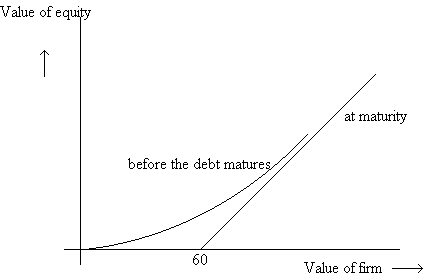Correspondingly, the value of the debt can be diagrammed as follows: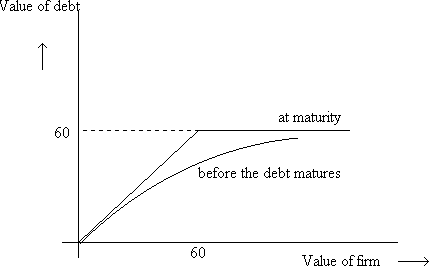#### Uses of options:

• Options can be used as insurance. For example, put options guarantee a floor value for the underlying asset.
• Investors can place bets on underlying stock return volatility by trading in stock call options.
• Investors (who are bearish on a stock) can place negative bets on the prospects for a stock by buying puts or selling calls.